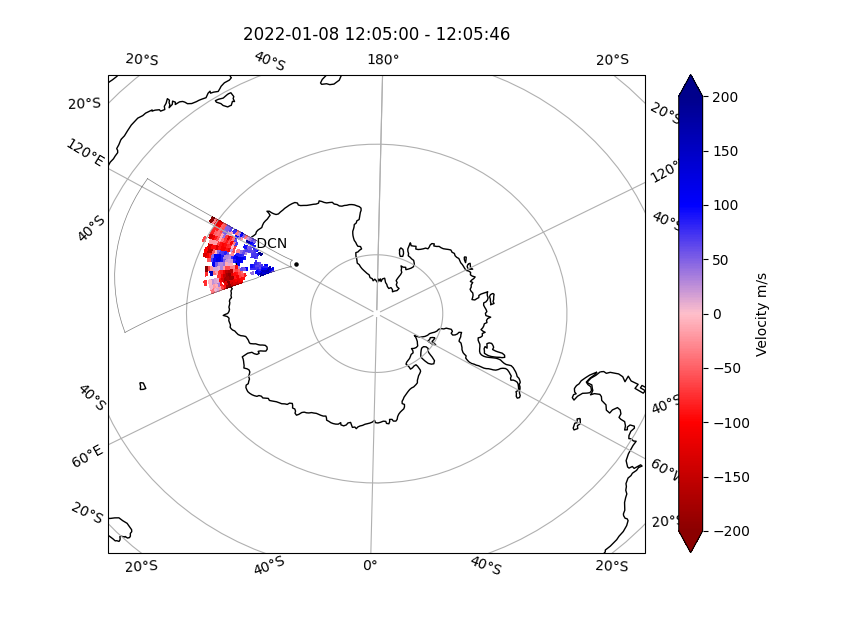# Fan plots

Fan plots are a way to visualise data from the entire scan of a SuperDARN radar.

All beams and ranges for a given parameter (such as line-of-sight velocity, backscatter power, etc) and a particular scan can be projected onto a polar format plot in AACGMv2 coordinates, or projected onto a geographic plot in geographic coordinates.

Warning

At present, AACGM coordinates cannot be plotted on a geographic projection.

The mapping of the range gate corners was based on rbpos in RST that is coded in `/pydarn/geo.py`. To get the coordinates read hardware docs.

### Basic usage

pyDARN and pyplot need to be imported, as well as any FITACF file needs to be read in:

``````import matplotlib.pyplot as plt
import pydarn

file = "path/to/fitacf/file"

``````

With the FITACF data loaded as a list of dictionaries (`fitacf_data` variable in above example), you may now call the `plot_fan` method. Make sure you tell it which scan (numbered from first recorded scan in file, counting from 1 or give it a `datetime` object for the scan at that time) you want using `scan_index`:

``````fanplot = pydarn.Fan.plot_fan(fitacf_data, scan_index=27,
colorbar_label='Velocity [m/s]')
plt.show()
``````

In this example, the 27th scan was plotted with the defaulted parameter being line-of-sight velocity: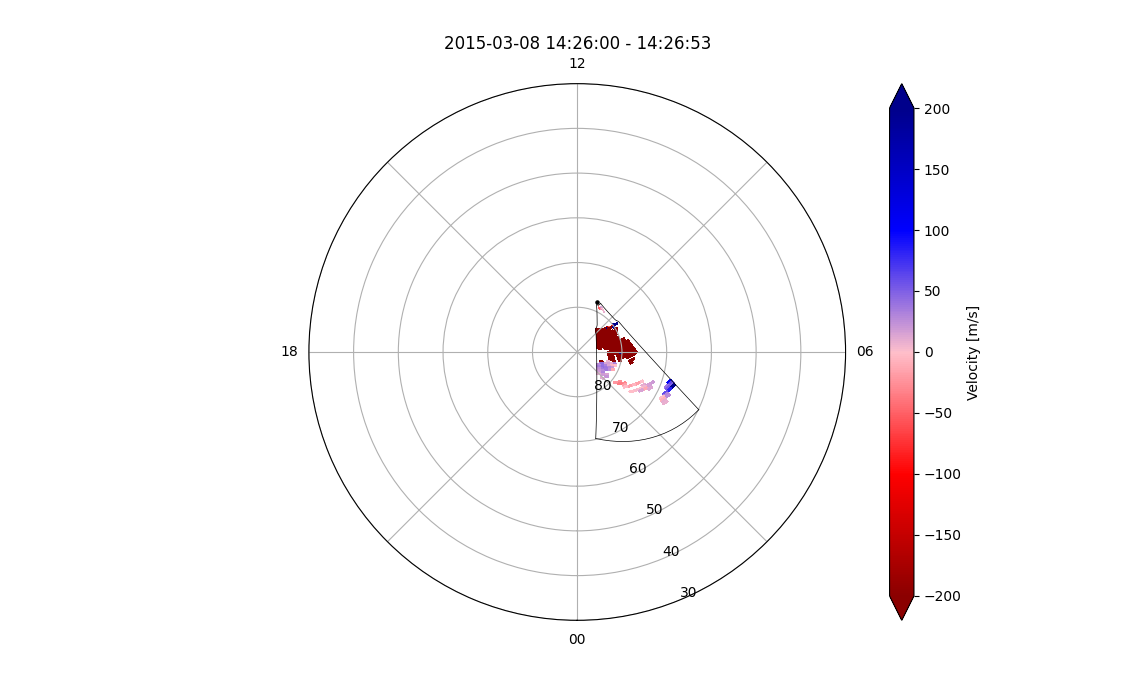You can also provide a `datetime` object to obtain a scan at a specific time:

``````pydarn.Fan.plot_fan(cly_data, scan_index=datetime(2015, 3, 8, 15, 26),
colorbar_label='Velocity [m/s]')
``````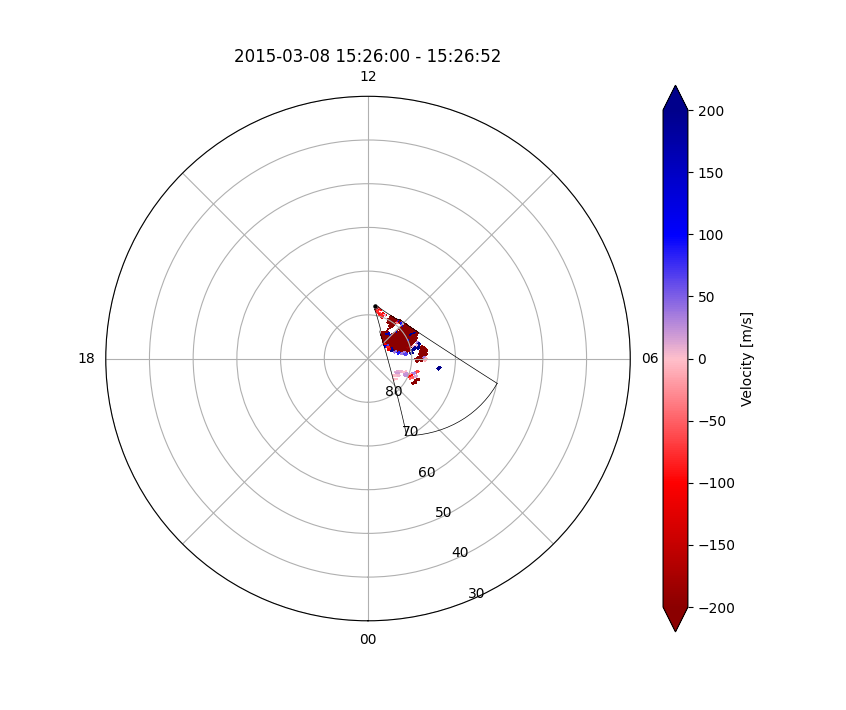Warning

Do not include seconds, typically scans are 1 minute long so seconds may end in a error with no data.

Default plots also do not show groundscatter as grey. Set it to true to colour groundscatter:

``````fanplot = pydarn.Fan.plot_fan(fitacf_data,
scan_index=27,
groundscatter=True)
plt.show()

``````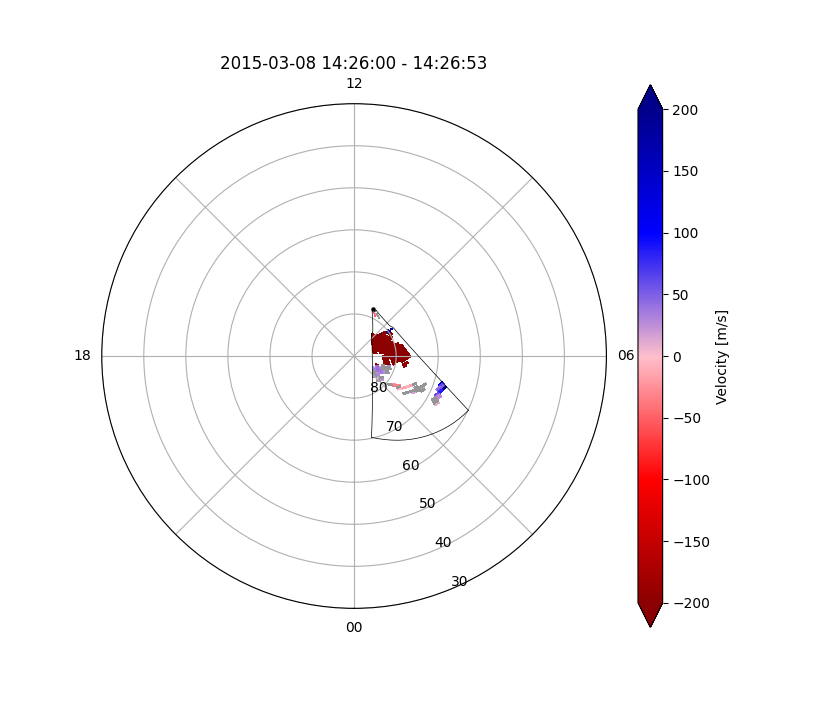You might have noticed that the variable `fanplot` in the examples above actually holds some information. This contains the AACGM latitude and longitude of the fan just plotted, as well as the data, ground scatter information, and datetime object. If you instead change `fanplot` to 5 separate variables, it will return the latitude, longitude, data, groundscatter and datetime info into seperate variables:

``````ax, lats, lons, data, grndsct, datetime = pydarn.Fan.plot_fan(fitacf_data,
scan_index=27)

lats.shape

``````

Which returns `>>>(76, 17)`, i.e. ranges x beams array of the latitude, and so on for the other variables. The groundscatter array is 0's and 1's, 1 being a range gate flagged as groundscatter.

In addition to line-of-sight velocity, you can choose one of three other data products to plot by setting `parameter=String name`:

Data product String name
Line of sight velocity (m/s) [Default] 'v'
Spectral width (m/s) 'w_l'
Elevation angle (degrees) 'elv'
Power (dB) 'p_l'

Here is a list of all the current options than can be used with `plot_fan`

Option Action
ax=(Axes Object) Matplotlib axes object than can be used for cartopy additions
scan_index=(int or datetime) Scan number or datetime, from start of records in file corresponding to channel if given
channel=(int or 'all') Specify channel number or choose 'all' (default = 'all')
parameter=(string) See above table for options
groundscatter=(bool) True or false to showing ground scatter as grey
ranges=(list) Two element list giving the lower and upper ranges to plot, grabs ranges from hardware file (default []
cmap=string A matplotlib color map string
zmin=(int) Minimum data value for colouring
zmax=(int) Maximum data value for colouring
colorbar=(bool) Set true to plot a colorbar (default: True)
colorbar_label=(string) Label for the colour bar (requires colorbar to be true)
title=(string) Title for the fan plot, default auto generated one based on input information
boundary=(bool) Set false to not show the outline of the radar FOV (default: True)
coords=(Coords) Coordinates for the data to be plotted in
projs=(Projs) Projections to plot the data on top of
kwargs ** Axis Polar settings. See polar axis

Note

For some control programs, the user may need to specify a channel integer as `'all'` will not correctly show the data. In other cases, the user may want to specify the channel and use an integer (N) for the `scan_index`. Be aware that this will show the data for the Nth scan of only the chosen channel, not that of the entire file.

`plot_fan` can concatenate with other plots including itself, here is an example of plotting two different radars with some of the above parameters:

``````import pydarn
from datetime import datetime
import matplotlib.pyplot as plt

cly_file = 'data/20150308.1400.03.cly.fitacf'
pyk_file = 'data/20150308.1401.00.pyk.fitacf'

pydarn.Fan.plot_fan(cly_data, scan_index=datetime(2015, 3, 8, 14, 4),
colorbar=False, fov_color='grey', line_color='blue',

pydarn.Fan.plot_fan(pyk_data, scan_index=datetime(2015, 3, 8, 14, 4),
colorbar_label='Velocity [m/s]', fov_color='grey',

#pydarn.Fan.plot_fov(66, datetime(2015, 3, 8, 15, 0), radar_label=True)
plt.show()
``````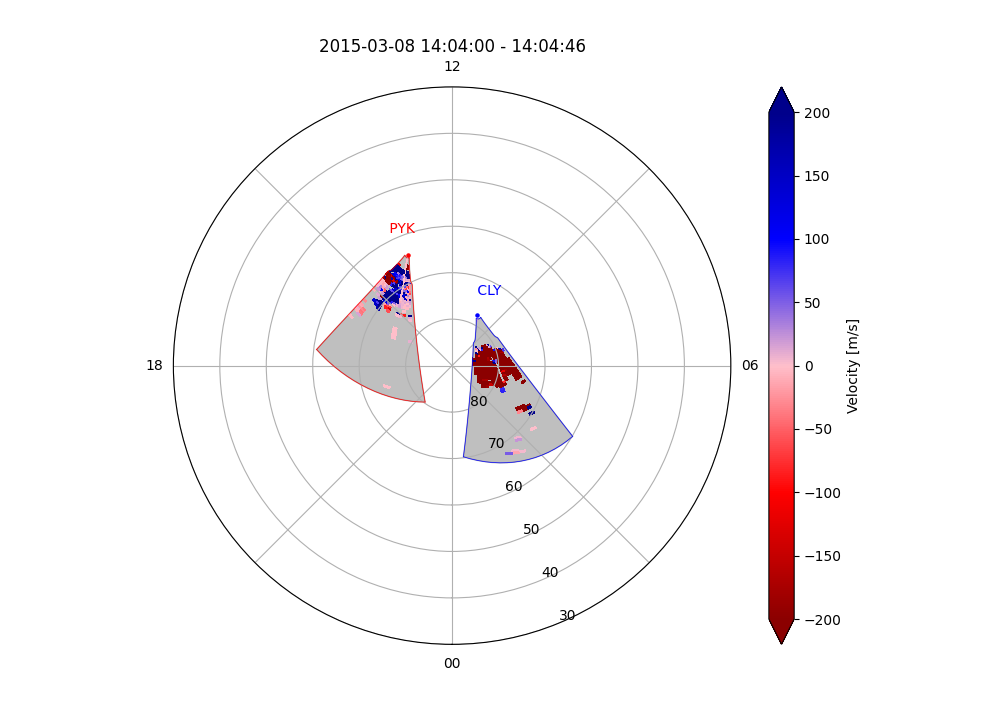Using cartopy to plot underlaid coastline map:

Warning

The cartopy coastlines mapping feature is currently only available for geographic projections in geographic coordinates. Expansion of this feature is coming soon!

``````ax, _, _, _, _ = pydarn.Fan.plot_fan(data, scan_index=5, radar_label=True, groundscatter=True, coords=pydarn.Coords.GEOGRAPHIC, projs=pydarn.     Projs.GEO, colorbar_label="Velocity m/s")
ax.coastlines()
plt.show()
``````